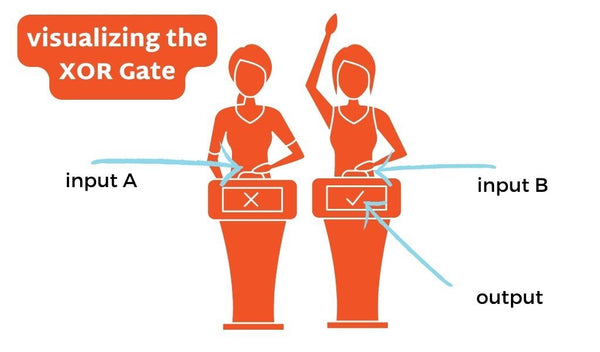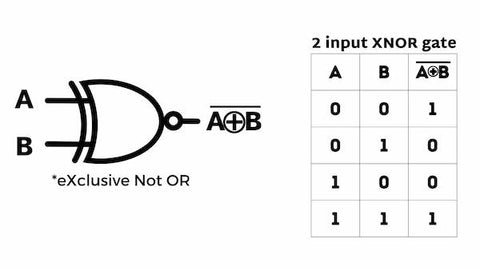# XOR Gate | Tutorial with examples, truth table, and downloadable assetsThe XOR Gate is a digital logic gate that outputs a high signal only when the input signals are different.

A fun example of an XOR Gate would be a game show buzzer. If two contestants buzz in, only one of them, the ﬁrst to buzz, will activate the circuit. The other contestant will be “locked out” from buzzing.XOR Gates are commonly used in digital circuits to perform arithmetic and data processing operations.

## The XOR Gate

The XOR module is a logic gate with two inputs and one output. The output of an XOR Gate is only HIGH (1) if either one, but not both, are high.

In the case of a XOR gate with more than two inputs, most of the time, an XOR gate will output a high signal if an odd number of the inputs are high.

The XOR is called the exclusive OR because it is similar to the OR gate, but it only outputs an HIGH if there is exclusively one input that is high. The XOR is great for projects in which the activation requires input alternation.

*Fyi, the following terms are used interchangeably
1 | HIGH | ON
O | LOW | OFF

In the truth table ON = 1 and OFF = 0. Let’s show you what that looks like.

## Truth Table of the XOR Gate

The truth table of an XOR gate summarizes its behavior for all possible input combinations. The following table shows the truth table for a 2-input XOR gate:Here are some real world applications of the XOR Gate.

## Applications of the XOR Gate

Here’s how we applied an XOR Gate to our book, Computer Engineering for Babies.Here are some other applications of the XOR Gate:

• Error detection and correction in digital data transmission
• Encryption and decryption in security systems
• Generation of control signals
• Creation of adders and subtractors in digital arithmetic circuits

Now that we know what they are used for, what are some of the different types

## Different types of XOR Gates

There are several types of XOR gates, including the standard 2-input XOR gate, as well as gates with more than two inputs.

• 2-Input XOR Gate
• 3-Input XOR Gate: This type of XOR gate has three inputs and one output. The output is high if an odd number of inputs are high, and low if an even number of inputs are high.
• 4-Input XOR Gate: This type of XOR gate has four inputs and one output. The output is high if an odd number of inputs are high, and low if an even number of inputs are high.
• eXclusive-Not-OR (XNOR Gate): This gate is sometimes referred to as the "equivalence" gate because its output is high if both inputs are the same (either both high or both low), and low if the inputs are different (one high and one low).## Advantages of using XOR Gates

XOR Gates are hugely versatile and can be combined with other logic gates to perform complex functions.

They are relatively simple and inexpensive to manufacture, making them a cost-effective solution for digital circuits. In addition to being cost-effective, XOR Gates can operate at high speeds, making them ideal for use in high-speed digital data processing systems.

## Disadvantages of using XOR Gates

There are also some disadvantages to using XOR gates in digital circuits. One of the main disadvantages is that they can generate significant amounts of electromagnetic interference (EMI), which can cause errors and signal degradation in nearby circuits.

The limited number of inputs can make them less suitable for certain types of digital processing applications that require more complex logic functions.

There are many ways to use a Gate in Computer Science and relating fields, here's how the XOR Gate compares to the others.

## Comparing XOR with Other Logic Gates

XOR gates have some similarities and differences when compared to other basic logic gates such as AND, OR, and NOT Gates.

Similarities:

• Like other basic logic gates, XOR gates operate on digital input signals and produce digital output signals.
• XOR gates are constructed using transistors and other electronic components, just like other logic gates.
• Like other basic logic gates, XOR gates can be combined in various ways to perform more complex functions.

Differences:

• Unlike AND Gates and OR Gates, which produce a high output signal when all or any of the input signals are high, XOR gates produce a high output signal only when the input signals are different.
• Unlike NOT gates, which have a single input and produce an inverted output signal, XOR gates can have multiple inputs and produce a non-inverted output signal.
• XOR gates have a limited number of inputs, while AND and OR gates can have any number of inputs.
• XOR gates can be more complex to design and troubleshoot than simpler logic gates, such as AND and OR gates.
• XOR gates have a tendency to generate electromagnetic interference (EMI), which can cause errors and signal degradation in nearby circuits.

## The XOR Gate in Digital Logic | Final thoughts

In summary, XOR gates have a unique output characteristic. They are often used in combination with other logic gates to create logic circuits that perform complex operations. Other logic gates, such as OR gates and NOT gates, have different output characteristics and correspond to different Boolean operations.

Did you notice the book images throughout this post? That’s our book, Computer Engineering for Babies, check it out!